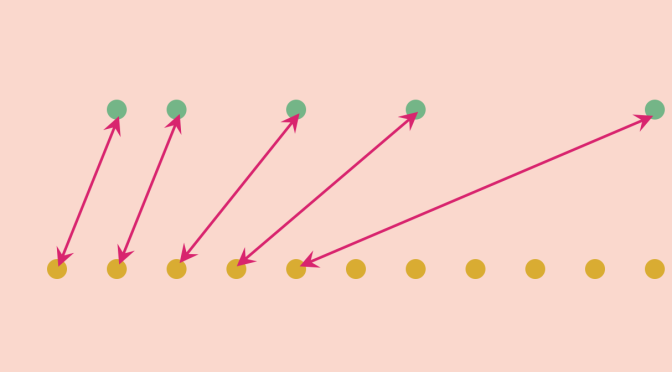Counterexamples around cardinality (part 1)

In mathematics, the cardinality of a set is a measure of the “number of elements of the set”. We denote by $$|A|$$ the cardinality of the set $$A$$. We say that $$A$$ and $$B$$ have the same cardinality if there exists a bijection from $$A$$ onto $$B$$. Such sets said to be equinumerous.

The finite sets satisfy the property that if $$A \subset B$$ and $$A$$ and $$B$$ have the same cardinality then $$A=B$$. For example take $$A=\{2,5,42\}$$. If $$A \subset B$$ and $$B$$ has more elements than $$A$$, a bijection $$f$$ from $$A$$ to $$B$$ cannot exist as an element in $$B \setminus \{f(2),f(5),f(42)\}$$ won’t have an inverse image under $$f$$.

This becomes false for infinite sets. Let’s give some examples.

Proper subsets of $$\mathbb N$$ equinumerous to $$\mathbb N$$

The set $$A$$ of the positive even integers is strictly included in $$\mathbb N$$: there exist odd positive integers! However, $$A$$ is equinumerous to $$\mathbb N$$. The map $$f : n \to 2n$$ is a bijection from $$A$$ onto $$\mathbb N$$.

For our second example, consider the set $$\mathbb P$$ of prime numbers. We recall that $$\mathbb P$$ is infinite. The proof is quite simple by contradiction. Suppose that there is only a finite number $$\{p_1, \dots, p_n\}$$ of prime numbers and consider the number $$N = p_1 p_2 \cdots p_n + 1$$. As a consequence of the fundamental theorem of arithmetic, a prime $$q$$ divides $$N$$. However $$q \notin \{p_1, \dots, p_n\}$$ as this would imply $$q$$ divides $$1$$. From there, we can define by induction a bijection from $$\mathbb N$$ onto $$\mathbb P$$ by defining $f(n)=\begin{cases} 2 & \text{for } n=1\\ \min (\mathbb P \setminus \{p_1, \dots, p_n\}) & \text{else } \end{cases}$

Proper subsets of $$\mathbb R$$ equinumerous to $$\mathbb R$$

Consider the map $\begin{array}{l|rcl} \tan : & (-\frac{\pi}{2}, \frac{\pi}{2}) & \longrightarrow & \mathbb R\\ & x & \longmapsto & \tan x \end{array}$ $$\tan$$ is continuous, strictly increasing and $$\lim\limits_{x \to \pm \frac{\pi}{2}} = \pm \infty$$. Hence, $$\tan$$ is a bijection from the proper subset $$(-\frac{\pi}{2}, \frac{\pi}{2})$$ of $$\mathbb R$$ onto $$\mathbb R$$.

Let’s consider a more amusing case! And take $\begin{array}{l|rcl} h : & \mathbb R & \longrightarrow & \mathbb R \setminus \{n \pi \ | n \in \mathbb N\}\\ & n \pi, \ n \in \mathbb N & \longmapsto & 2n+1\\ & n \in \mathbb N & \longmapsto & 2n\\ & x & \longmapsto & x \text{ else} \end{array}$ One can verify that $$h$$ is a bijection. However the image of $$\mathbb R$$ under $$h$$ excludes all reals from the set $$\{n \pi \ | n \in \mathbb N\}$$.

Can we follow on this path and find a bijection from $$\mathbb R$$ onto the rational numbers? The answer is negative and I will come back later on on this question.